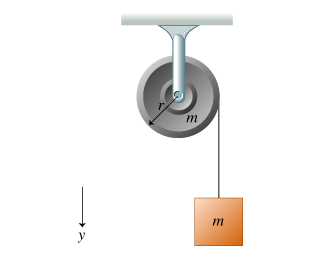# Problem: A string is wrapped around a uniform solid cylinder of radius, r as shown in the figure (Figure 1). The cylinder can rotate freely about its axis. The loose end of the string is attached to a block. The block and cylinder each have mass m. Note that the positive y direction is downward and counterclockwise torques are positive.Find the magnitude α of the angular acceleration of the cylinder as the block descends.Express your answer in terms of the cylinder's radius r and the magnitude of the acceleration due to gravity g.

###### FREE Expert Solution

Newton's second law:

$\overline{){\mathbf{\Sigma }}{\mathbf{F}}{\mathbf{=}}{\mathbf{m}}{\mathbf{a}}}$

Relationship between angular acceleration, α, and linear acceleration, a:

$\overline{){\mathbf{a}}{\mathbf{=}}{\mathbf{r}}{\mathbf{·}}{\mathbf{\alpha }}}$

Torque:

$\overline{){\mathbf{\tau }}{\mathbf{=}}{\mathbf{r}}{\mathbf{·}}{\mathbf{F}}}$

Torque and angular acceleration:

$\overline{){\mathbf{\tau }}{\mathbf{=}}{\mathbf{I}}{\mathbf{·}}{\mathbf{\alpha }}}$

Moment of inertia of a cylinder:

$\overline{){\mathbit{I}}{\mathbf{=}}\frac{\mathbf{1}}{\mathbf{2}}{\mathbf{m}}{{\mathbf{r}}}^{{\mathbf{2}}}}$

where I is the moment of inertia.

92% (95 ratings)###### Problem Details

A string is wrapped around a uniform solid cylinder of radius, r as shown in the figure (Figure 1). The cylinder can rotate freely about its axis. The loose end of the string is attached to a block. The block and cylinder each have mass m. Note that the positive y direction is downward and counterclockwise torques are positive.Find the magnitude α of the angular acceleration of the cylinder as the block descends.

Express your answer in terms of the cylinder's radius r and the magnitude of the acceleration due to gravity g.